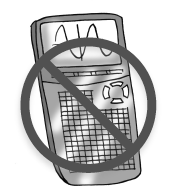### Home > INT1 > Chapter A > Lesson A.1.2 > ProblemA-25

A-25.

Write $\left(1.75 × 10^{20}\right)\left(6.01 × 10^{14}\right)$ in scientific notation without using a calculator. Then check your answer with your calculator. Write down exactly what your calculator displayed.Use the Commutative Property of Multiplication to rearrange the terms, then simplify: $\left(1.75\right)\left(6.01\right) × \left(10^{20}\right)\left(10^{14}\right)$.

Be sure your answer is in the format $a \times 10^{n}$ and that $1 ≤ a < 10$.

For more help, see Checkpoint 5A.